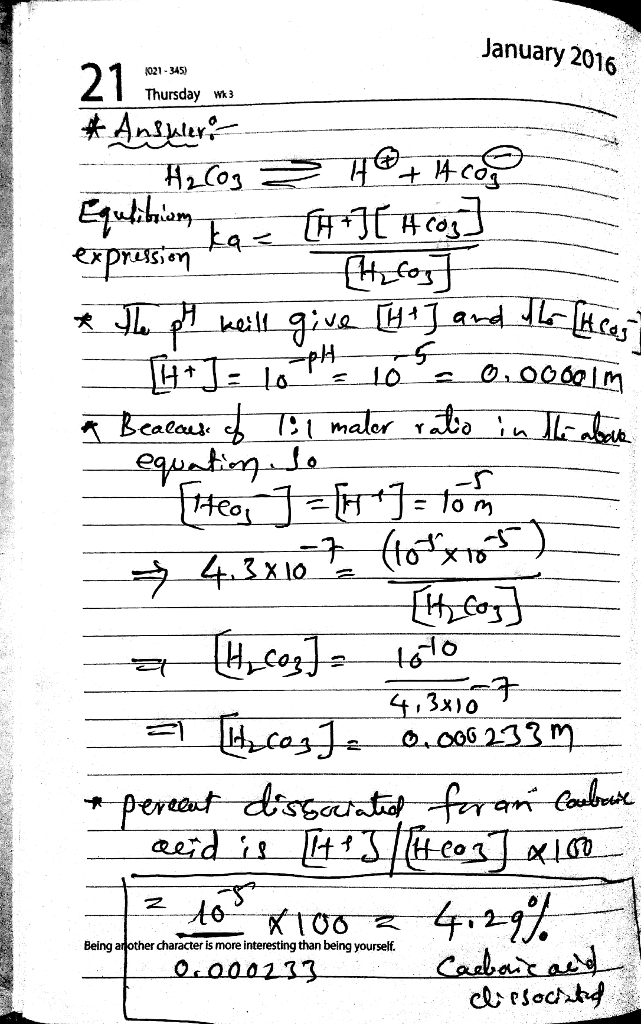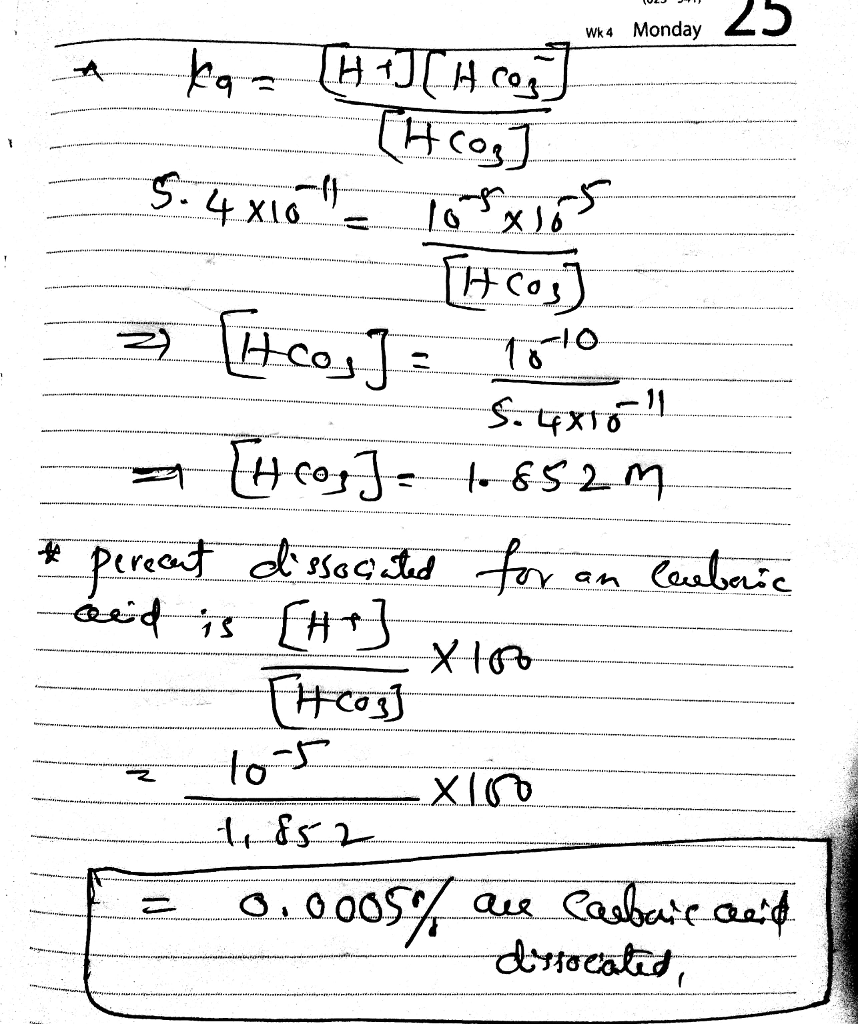# Homework Solution: If pH=5, how much carbonic acid has dissociated? CO_2 + H_2O rightarrow…

If pH=5, how much carbonic acid has dissociated? CO_2 + H_2O rightarrow H_2CO_3 H_2CO_3 right harpoon over left harpoon H^+ + HCO_3^- If pH = 5, how much H_2CO_3 has dissociated? Ka_1 = 4.3 times 10^-7 Ka_2 = 5.6 times 10^-11